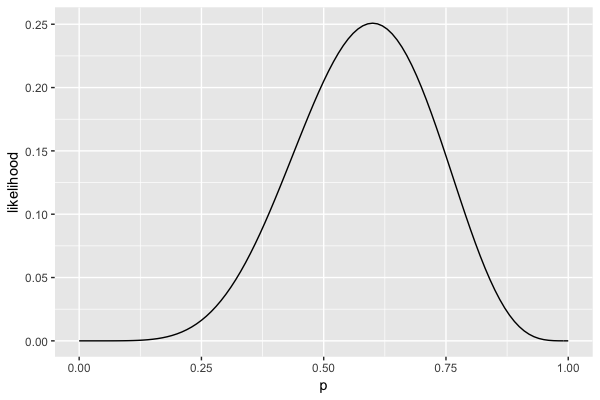# Interpreting the likelihood function

In the previous exercise you approximated the likelihood function shown below. The likelihood highlights the relative compatibility of different possible values of $$p$$, your underlying election support, with the observed poll in which $$X = 6$$ of $$n = 10$$ voters supported you. Specifically, the height of the likelihood function at any given value of $$p$$ reflects the relative plausibility of observing these particular polling data if your underlying support were equal to $$p$$. Thus which of the following two scenarios is more compatible with the poll data?

• Scenario 1: your underlying support $$p$$ is around 45%.
• Scenario 2: your underlying support $$p$$ is around 55%.